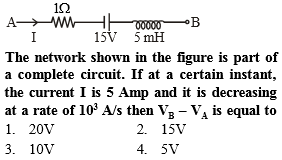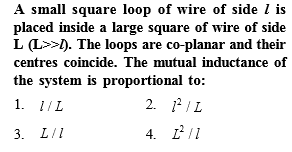# NEET Physics Electromagnetic Induction Questions Solved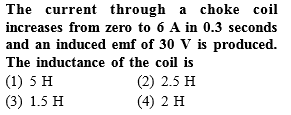Complete Question Bank + Test Series
Complete Question Bank

Difficulty Level: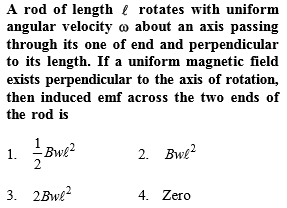Complete Question Bank + Test Series
Complete Question Bank

Difficulty Level: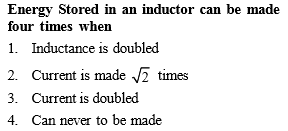Complete Question Bank + Test Series
Complete Question Bank

Difficulty Level:

A coil wire of a certain radius has 600 turns and a self-inductance of 36 mH. The self-inductance of a second similar coil of 500 turns will be

1.  25 mH

2.  50mH

3.  108mH

4.  75mH

Complete Question Bank + Test Series
Complete Question Bank

Difficulty Level: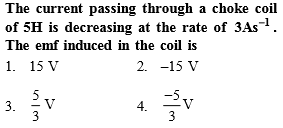Complete Question Bank + Test Series
Complete Question Bank

Difficulty Level:

If all linear dimensions of an inductor are tripled, then self inductance will become (keeping total number of turns per unit length constant)

1.  3 times

2.  9 times

3.  27 times

4. $\frac{1}{3}$ times

Complete Question Bank + Test Series
Complete Question Bank

Difficulty Level: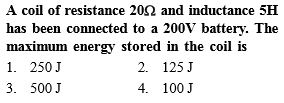Complete Question Bank + Test Series
Complete Question Bank

Difficulty Level: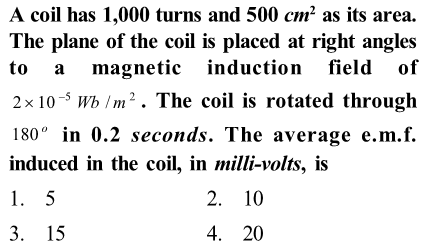Complete Question Bank + Test Series
Complete Question Bank

Difficulty Level: